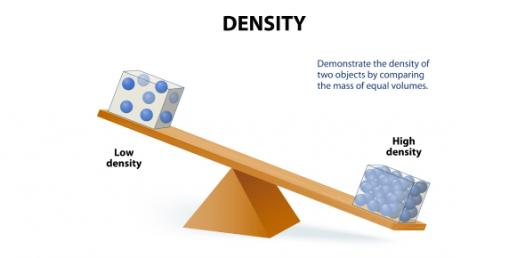# Battles Density Quiz

5 Questions | Attempts: 3865
ShareSettings• 1.
An object with a density of 0.85 g/cm3 is dropped into each of the two beakers shown below. What will happen to the object in each case?
• A.

It will sink in both beakers.

• B.

It will float in beaker 1 and sink in beaker 2.

• C.

It will sink in beaker 1 and float in beaker 2.

• D.

It will float in both beakers.

• 2.
Objects 1 and 2 float as shown in the beaker below. The density of the liquid in the beaker is 3.2 g/mL. Which of the densities given below are possible values for the densities of the two objects?
• A.

Density of object 1 = 4.4 g/cm3 Density of object 2 = 4.0 g/cm3

• B.

Density of object 1 = 3.0 g/cm3 Density of object 2 = 1.8 g/cm3

• C.

Density of object 1 = 1.6 g/cm3 Density of object 2 = 2.9 g/cm3

• D.

Density of object 1 = 2.5 g/cm3 Density of object 2 = 3.8 g/cm3

• 3.
Identical objects are placed in Beaker A and in Beaker B. The objects float as shown in the diagrams below. What can you conclude about the liquid in each of the beakers?
• A.

Both liquids are less dense than the object.

• B.

The liquid in Beaker A has a greater density than the objects. The liquid in Beaker B is less dense than the objects.

• C.

The liquid in Beaker A has a greater density than the liquid in Beaker B.

• D.

The liquid in Beaker B has a greater density than the liquid in Beaker A.

• 4.
Objects 1 and 2 are placed in the beaker below. The density of the liquid in the beaker is 2.0 g/mL. Which of the densities given below are possible values for the densities of the two objects?
• A.

Density of object 1 = 0.8 g/cm3 Density of object 2 = 3.0 g/cm3

• B.

Density of object 1 = 3.0 g/cm3 Density of object 2 = 0.8 g/cm3

• C.

Density of object 1 = 3.8 g/cm3 Density of object 2 = 3.0 g/cm3

• D.

Density of object 1 = 1.5 g/cm3 Density of object 2 = 0.8 g/cm3

• 5.
An object with unknown density is tested in two beakers. Beaker 1 contains liquid with a density of 1.5 g/mL, and Beaker 2 contains liquid with a density of 2.0 g/mL. The object sinks in both beakers. Which of the following statements about the object's density is true?
• A.

The object's density is less than 1.5 g/cm3.

• B.

The object's density is exactly 1.5 g/cm3.

• C.

The object's density is greater than 1.5 g/cm3 and less than 2.0 g/cm3.

• D.

The object's density is greater than 2.0 g/cm3.

## Related TopicsBack to top
×

Wait!
Here's an interesting quiz for you.